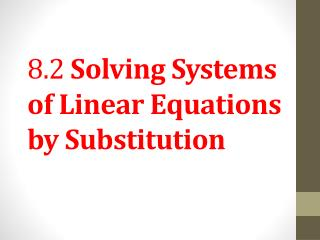DownloadDownload Presentation8.2 Solving Systems of Linear Equations by Substitution

# 8.2 Solving Systems of Linear Equations by Substitution

Download Presentation## 8.2 Solving Systems of Linear Equations by Substitution

- - - - - - - - - - - - - - - - - - - - - - - - - - - E N D - - - - - - - - - - - - - - - - - - - - - - - - - - -
##### Presentation Transcript

1. 8.2 Solving Systems of Linear Equations by Substitution

2. Objective 1 Solve linear systems by substitution. Slide 8.2-3

3. Solve linear systems by substitution. Graphing to solve a system of equations has a serious drawback. It is difficult to find an accurate solution, such as from a graph. One algebraic method for solving a system of equations is the substitution method. This method is particularly useful for solving systems in which one equation is already solved, or can be solved quickly, for one of the variables. Slide 8.2-4

4. CLASSROOM EXAMPLE 1 Using the Substitution Method Solve the system by the substitution method. Solution: The solution set found by the substitution method will be the same as the solution found by graphing. The solution set is the same; only the method is different. A system is not completely solved until values for both x and y are found. Slide 8.2-5

5. CLASSROOM EXAMPLE 2 Using the Substitution Method Solve the system by the substitution method. Solution: Be careful when you write the ordered-pair solution of a system. Even though we found y first, the x-coordinate is always written first in the ordered pair. Slide 8.2-6

6. Solve linear systems by substitution. (cont’d) Solving a Linear System by Substitution Step 1:Solve one equation for either variable.If one of the variables has coefficient 1 or −1, choose it, since it usually makes the substitution method easier. Step 2:Substitute for that variable in the other equation. The result should be an equation with just one variable. Step 3:Solvethe equation fromStep 2. Step 4:Substitutethe result from Step 3 into the equation from Step 1 to find the value of the other variable. Step 5:Checkthe solution in both of the original equations. Then write the solution set. Slide 8.2-7

7. CLASSROOM EXAMPLE 3 Using the Substitution Method Use substitution to solve the system. Solution: Slide 8.2-8

8. Objective 2 Solve special systems by substitution. Slide 8.2-9

9. Solve special systems by substitution. Recall from Section 8.1 that systems of equations with graphs that are parallel lines have no solution. Systems of equations with graphs that are the same line have an infinite number of solutions. Slide 8.2-10

10. CLASSROOM EXAMPLE 4 Solving an Inconsistent System by Substitution Use substitution to solve the system. Solution: Since the statement is false, the solution set is Ø. It is a common error to give “false” as the solution of an inconsistent system. The correct response is Ø. Slide 8.2-11

11. CLASSROOM EXAMPLE 5 Solving a System with Dependent Equations by Substitution Solve the system by the substitution method. Solution: Since the statement is true every solution of one equations is also a solution to the other, so the system has an infinite number of solutions and the solution set is {(x,y)|x + 3y = −7}. It is a common error to give “true” as the solution of a system of dependent equations. Remember to give the solution set in set-builder notation using the equation in the system that is in standard form with integer coefficients that have no common factor (except 1). Slide 8.2-12

12. Objective 3 Solve linear systems with fractions and decimals by substitution. Slide 8.2-13

13. CLASSROOM EXAMPLE 6 Using the Substitution Method with Fractions as Coefficients Solve the system by the substitution method. Solution: Slide 8.2-14

14. CLASSROOM EXAMPLE 7 Using the Substitution Method with Decimals as Coefficients Solve the system by the substitution method. Solution: Slide 8.2-15